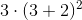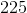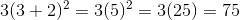# High School Math : High School Math

## Example Questions

### Example Question #21 : High School Math

Evaluate the following expression: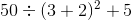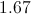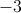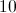Explanation:

To solve this problem, we must follow the order of operations. That is: Parentheses, Exponent, Multiply, Divide, Addition, Subtraction (PEMDAS).

First, we evaluate the parentheses: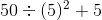Next, we evaluate the exponents: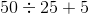Next, we complete the multiplication and division from the left to the right of the expression: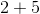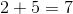### Example Question #22 : High School Math

Evaluate the following expression: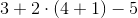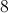Explanation:

To solve this problem, we must follow the order of operations. That is: Parentheses, Exponent, Multiply, Divide, Addition, Subtraction (PEMDAS).

First, we evaluate the parentheses: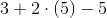Next, we complete the multiplication: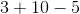Finally, we evaluate the addition and subtraction from left to right in the expression: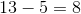### Example Question #23 : High School Math

Evaluate the following expression: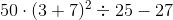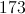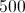Explanation:

To solve this problem, we must follow the order of operations. That is: Parentheses, Exponent, Multiply, Divide, Addition, Subtraction (PEMDAS).

First, we evaluate the parentheses: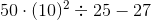Next, we evaluate the exponents: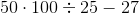Next, following PEMDAS, we evaluate the multiplication and division from left to right in the expression: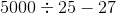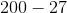Finally, we evaluate the subtraction: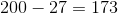### Example Question #2 : Order Of Operations

Solve the following problem: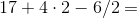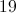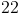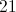Explanation:

First, work from left to right completing multiplication and division, then work from left to right completing addition and subtraction.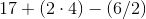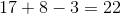### Example Question #1 : Multiplication And Division

Express in degrees: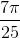radians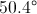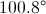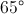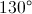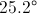Explanation:

Since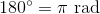, we can convert as follows: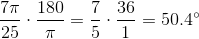### Example Question #2 : Multiplication And Division

Suppose you know the values ofand, and you want to evaluate the expression: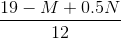In which order would you carry out the four operations in the expression?

Explanation:

A fraction bar in an expression acts as both a division symbol and a grouping symbol, so we evaluate the numerator first. Within the numerator, there is a multplication, a subtraction, and an addition, so, by order of operations, we multiply first. Addition and subtraction are carried out right to left; the subtraction is left of the addition, so we subtract next, then add. Finally, we divide the numerator by the denominator.

In summary: Multiply, subtract, add, divide

### Example Question #3 : Order Of Operations

Suppose you know the value of, and you want to evaluate the expression: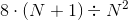In which order would you carry out the four operations in the expression?

Explanation:

By order of operations, always carry out any operations within parentheses first; this is the addition.  This removes the parentheses; what remains is a square, a multiplication, and a division. Since there are no more grouping symbols, square next. The multiplication is done next, as multiplications and divisions are performed in left-to-right order.

In summary: Add, square, multiply, divide

### Example Question #4 : Order Of Operations

Suppose you know the value of, and you want to evaluate the expression: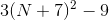In which order would you carry out the four operations in the expression?

Explanation:

By order of operations, always carry out any operations within parentheses first; this is the addition. This removes the parentheses; what remains is a square, a multiplication, and a subtraction. This is the correct order in the absence of grouping symbols.

In summary: Add, square, multiply, subtract

### Example Question #5 : Order Of Operations

Simplify the expression.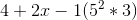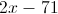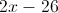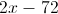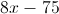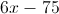Explanation:

The order of operations is parenthesis, exponents, multiplication, division, addition, subtraction (PEMDAS).First, we will evaluate the parentheses. Within the parentheses, we need to solve the exponent, then multiply,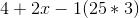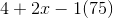Now that the parenthesis is evaluated, we need to multiply.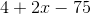Finally, we add and subtract. We can arrange the terms in any order.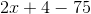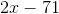### Example Question #24 : High School Math

Evaluate the following expression: